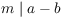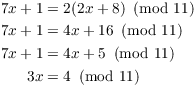# Modular Arithmetic

Definiton. Let a, b, and m be integers. a is congruent to b mod m if; that is, ifNotation:means that a is congruent to b mod m. m is called the modulus of the congruence; I will almost always work with positive moduli.

Note thatif and only if. Thus, modular arithmetic gives you another way of dealing with divisibility relations.

For example:because.because.

Proposition. Congruence mod m is an equivalence relation:

(a) ( Reflexivity)for all a.

(b) ( Symmetry) If, then.

(c) ( Transitivity) Ifand, then.

Proof. Since, it follows that.

Suppose. Then, so. Hence,.

Supposeand. Then there are integers j and k such thatAdd the two equations:This implies that.Example. (a) List the elements of the equivalence classes relative to congruence mod 3.

(b) Using 0, 1, and 2 to represent these equivalence classes, construct addition and multiplication tables mod 3.

(a) The equivalence classes are the 3 congruence classes:Each integer belongs to exactly one of these classes. Two integers in a given class are congruent mod 3.

(If you know some group theory, you probably recognize this as constructingfrom.)(b)For example,, becauseas integers, and the congruence class of 3 is represented by 0. Likewise,as integers, and the congruence class of 4 is represented by 1.I could have chosen different representatives for the classes --- say 3, -4, and 4. A choice of representatives, one from each class, is called a complete system of residues mod 3. But working mod 3 it's natural to use the numbers 0, 1, and 2 as representatives --- and in general, if I'm working mod n, the obvious choice of representatives is the set. This set is called the standard residue system mod n, and it is the set of representatives I'll usually use. Thus, the standard residue system mod 6 is.

Theorem. Supposeand. Then:

(a)and.

(b).

Proof. I'll prove the first congruence as an example. Supposeand. Thenandfor some, soThis implies that.Example. Solve the congruenceThere are no "fractions" mod 11. I want to divide by 3, and to do this I need to multiply by the multiplicative inverse of 3. So I need a number k such that. A systematic way of finding such a number is to use the Extended Euclidean algorithm. In this case, I just use trial and error. Obviously,andwon't work, so I'll start at:Thus, I need to multiply the equation by 4:Definition. x and y are multiplicative inverses mod n if.

Notation:or. Do not use fractions.

Example. (a) Find.

(b) Prove that 6 does not have a multiplicative inverse mod 8.

(a), so.(b) Suppose. ThenThis contradiction shows that 6 does not have a multiplicative inverse mod 8.Example. Reduceto a number in.Example. Reduceto a number in., soExample. Show that if p is prime, thenBy the Binomial Theorem,A typical coefficientis divisible by p for. So going mod p, the only terms that remain areand.

For exampleThe result is not true if the modulus is not prime. For example,Contact information

Copyright 2018 by Bruce Ikenaga